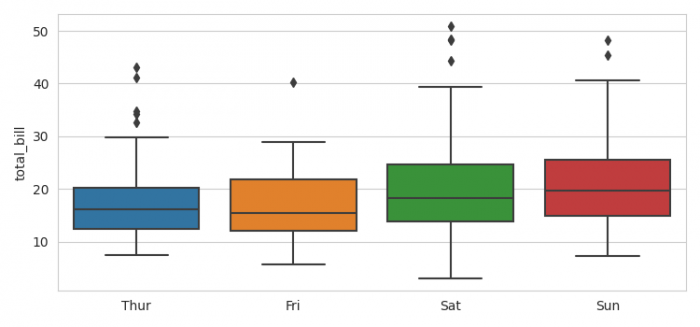# How to remove or hide X-axis labels from a Seaborn / Matplotlib plot?

To remove or hide X-axis labels from a Seaborn/Matplotlib plot, we can take the following steps −

• Set the figure size and adjust the padding between and around the subplots.

• Use sns.set_style() to set an aesthetic style for the Seaborn plot.

• Load an example dataset from the online repository (requires Internet).

• To hide or remove X-axis labels, use set(xlabel=None).

• To display the figure, use show() method.

## Example

from matplotlib import pyplot as plt
import seaborn as sns

plt.rcParams["figure.figsize"] = [7.50, 3.50]
plt.rcParams["figure.autolayout"] = True

sns.set_style("whitegrid")
plt.show()